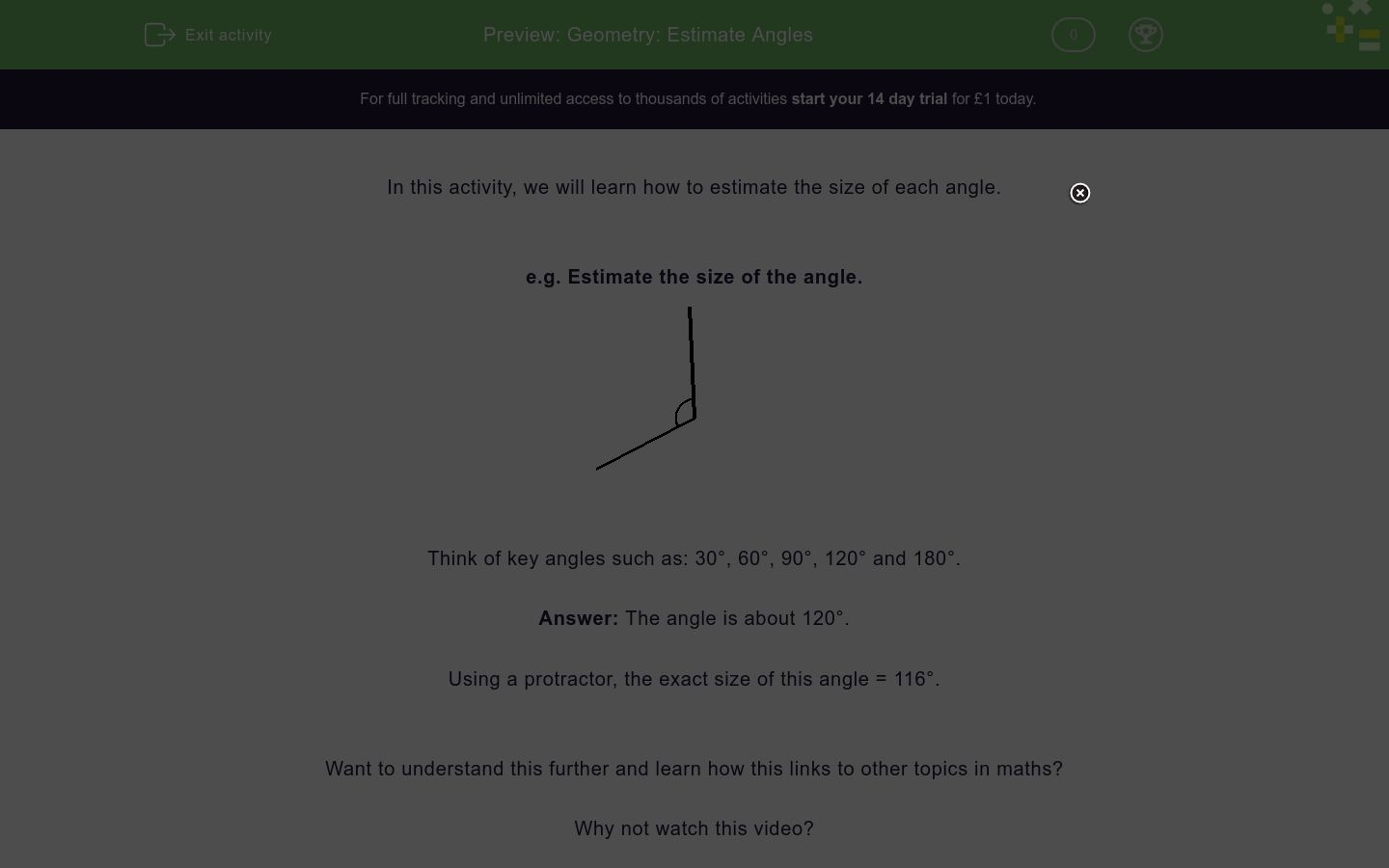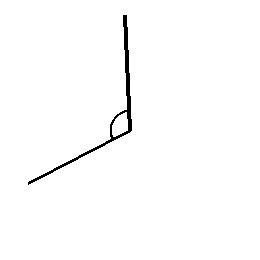# Geometry: Estimate Angles

In this worksheet, students use their knowledge to estimate an angle. Then they measure the angle to see how close they were.Key stage:  KS 2

Curriculum topic:   Geometry: Properties of Shapes

Curriculum subtopic:   Know Angles and Degrees

Difficulty level:### QUESTION 1 of 10

In this activity, we will learn how to estimate the size of each angle.

e.g. Estimate the size of the angle.Think of key angles such as: 30°, 60°, 90°, 120° and 180°.

Using a protractor, the exact size of this angle = 116°.

Want to understand this further and learn how this links to other topics in maths?

Why not watch this video?

Estimate the size of the angle.109

135

164

49

Estimate the size of the angle.98

143

185

275

Estimate the size of the angle.58

85

285

138

Estimate the size of the angle.40

125

285

89

Estimate the size of the angle.145

56

125

89

Estimate the size of the angle.185

56

115

254

Estimate the size of the angle.165

117

45

224

Estimate the size of the angle.50

90

150

120

Estimate the size of the angle.160

30

90

109

Estimate the size of the angle.100

90

56

85

• Question 1

Estimate the size of the angle.109
EDDIE SAYS
The angle is obtuse, so it's more than 90. A protractor can be used for checking.
• Question 2

Estimate the size of the angle.143
EDDIE SAYS
The angle is obtuse, so it's more than 90. Use a protractor to check.
• Question 3

Estimate the size of the angle.138
EDDIE SAYS
The angle is obtuse, so it's more than 90. Use a protractor to check.
• Question 4

Estimate the size of the angle.89
EDDIE SAYS
The angle looks close to 90, or a right angle. Use a protractor to check.
• Question 5

Estimate the size of the angle.56
EDDIE SAYS
The angle is acute, so it's less than 90. Use a protractor to check.
• Question 6

Estimate the size of the angle.115
EDDIE SAYS
The angle is obtuse, so it's more than 90. Use a protractor to check.
• Question 7

Estimate the size of the angle.117
EDDIE SAYS
The angle is obtuse, so it's more than 90. Use a protractor to check.
• Question 8

Estimate the size of the angle.120
EDDIE SAYS
The angle is obtuse, so it's more than 90. Use a protractor to check.
• Question 9

Estimate the size of the angle.109
EDDIE SAYS
The angle is obtuse, so it's more than 90. A protractor can be used for checking.
• Question 10

Estimate the size of the angle.56
EDDIE SAYS
The angle is acute, so it's less than 90. Use a protractor to check.
---- OR ----

Sign up for a £1 trial so you can track and measure your child's progress on this activity.

### What is EdPlace?

We're your National Curriculum aligned online education content provider helping each child succeed in English, maths and science from year 1 to GCSE. With an EdPlace account you’ll be able to track and measure progress, helping each child achieve their best. We build confidence and attainment by personalising each child’s learning at a level that suits them.

Get started